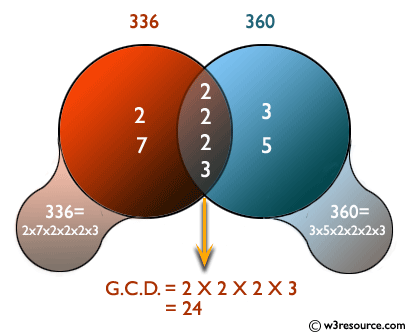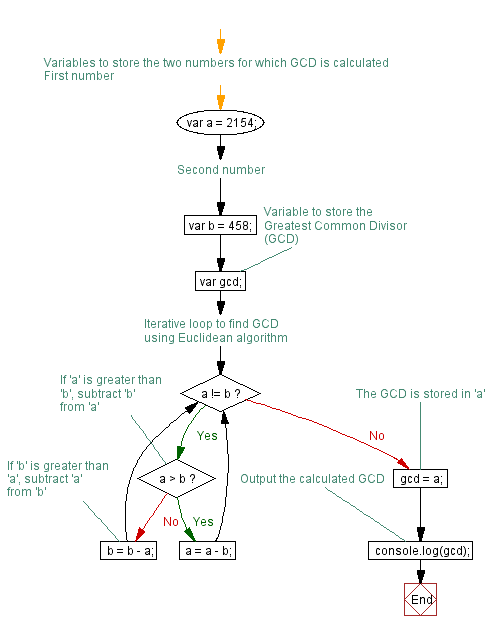# JavaScript: Compute the greatest common divisor (GCD) of two positive integers

## JavaScript Conditional Statement and loops: Exercise-11 with Solution

Write a JavaScript program to compute the greatest common divisor (GCD) of two positive integers.

Pictorial Presentation:Sample Solution:-

HTML Code:

``````<!DOCTYPE html>
<html>
<meta charset="utf-8">
<title>compute the greatest common divisor (GCD) of two positive integers</title>
<body>

</body>
</html>
```
```

JavaScript Code:

``````var a = 2154; //First number
var b = 458;  //Second number
var gcd;
while (a!=b)
{
if (a>b)
{
a = a -b;
}
else
{
b = b - a;
}
}
gcd = a;
console.log(gcd);
```
```

Sample Output:

```2
```

Flowchart:Live Demo:

See the Pen javascript-conditional-statements-and-loops-exercise-11 by w3resource (@w3resource) on CodePen.

Improve this sample solution and post your code through Disqus

What is the difficulty level of this exercise?

Test your Programming skills with w3resource's quiz.

﻿

## JavaScript: Tips of the Day

Creates an array of elements, ungrouping the elements in an array produced by zip and applying the provided function

Example:

```const tips_unzip = (arr, fn) =>
arr
.reduce(
(acc, val) => (val.forEach((v, i) => acc[i].push(v)), acc),
Array.from({
length: Math.max(...arr.map(x => x.length))
}).map(x => [])
)
.map(val => fn(...val));

console.log(tips_unzip([[2, 15, 200], [3, 25, 300]], (...args) => args.reduce((acc, v) => acc + v, 0)));
```

Output:

```[5, 40, 500]
```